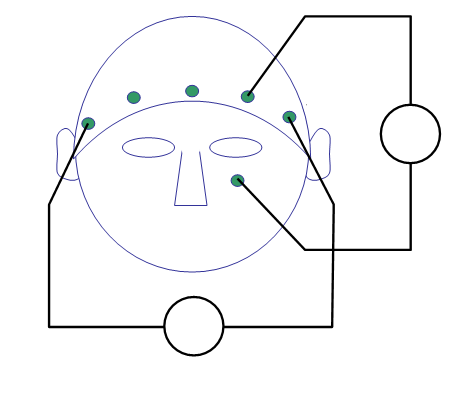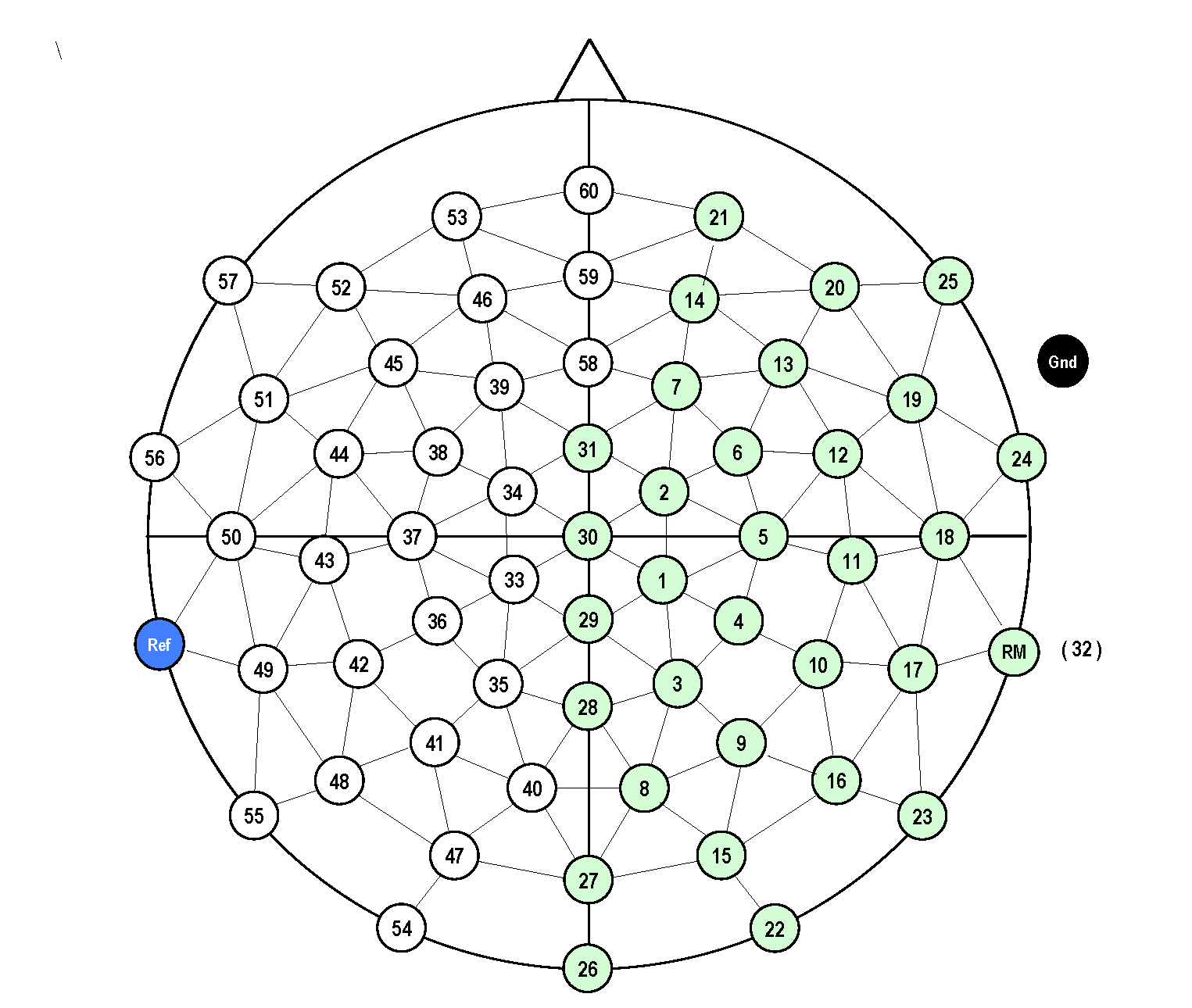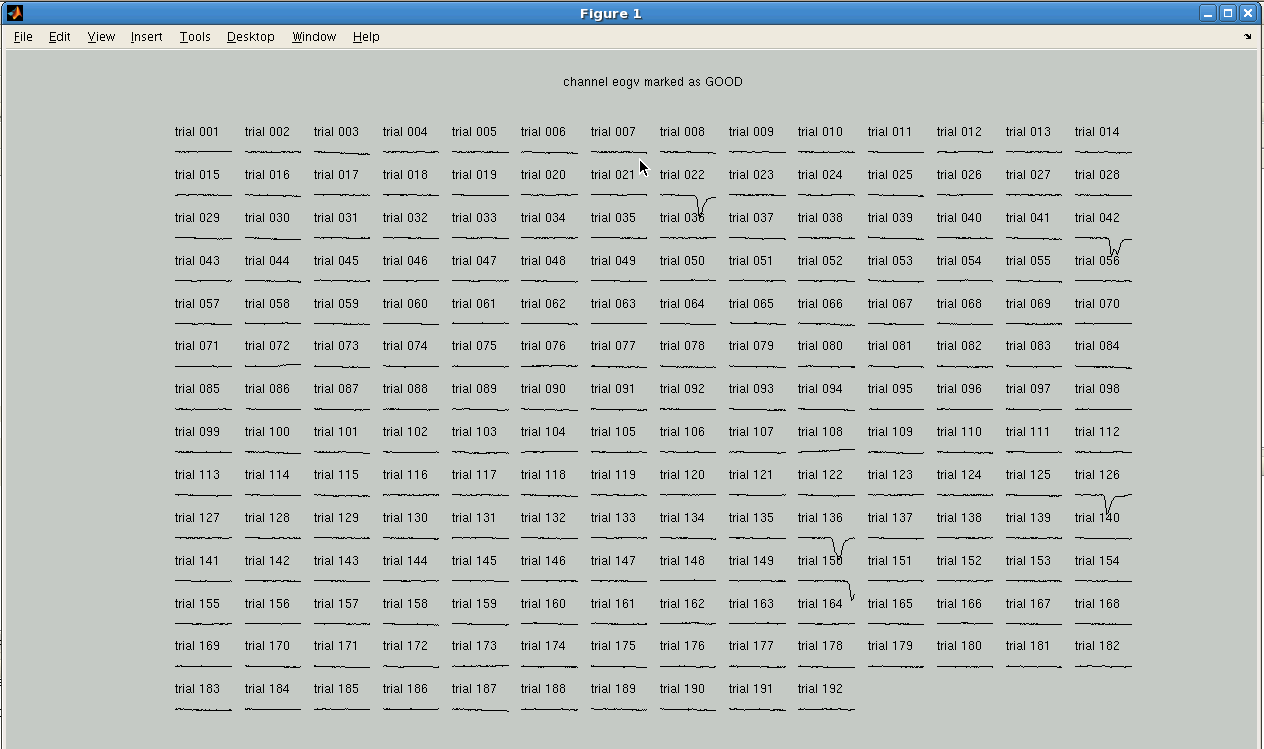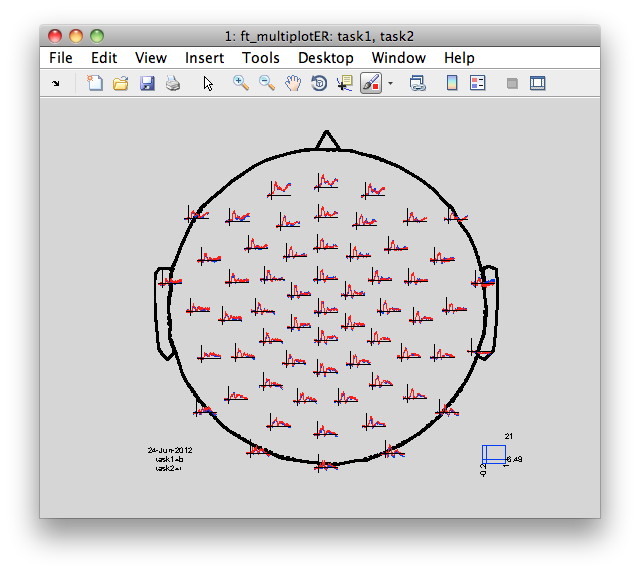# Preprocessing of EEG data and computing ERPs

## Background

In FieldTrip the preprocessing of data refers to the reading of the data, segmenting the data around interesting events such as triggers, temporal filtering and optionally rereferencing. The ft_preprocessing function takes care of all these steps, i.e., it reads the data and applies the preprocessing options.

There are largely two alternative approaches for preprocessing, which differ in the amount of memory required in the order of the individual analysis steps. The first approach is to read all data from the file into memory, apply filters, and subsequently cut the data into interesting segments. The second approach is to first identify the interesting segments, read those segments from the data file and apply the filters to those segments only. The remainder of this tutorial explains the second approach. This is mainly motivated by the historical development of FieldTrip: in the early days of the toolbox, computer memory as a more limiting factor than nowadays. Also, note, that some (older) datasets may have the data already represented on disk as epoched, which prohibits the treatment of the data as a single continuous data matrix. The approach for reading and filtering continuous data and segmenting afterwards is explained in another tutorial.

Preprocessing involves several steps, including identifying individual trials from the dataset, filtering and artifact rejections. This tutorial covers how to identify trials using the trigger signal. Defining data segments of interest can be done according to a specified trigger channel or according to your own criteria when you write your own trial function. Examples for both ways are described in this tutorial, and both ways depend on ft_definetrial.

The output of ft_definetrial is a configuration structure containing the field cfg.trl. This is a matrix representing the relevant parts of the raw datafile which are to be selected for further processing. Each row in the `trl` matrix represents a single epoch-of-interest, and the `trl` matrix has at least 3 columns. The first column defines (in samples) the beginpoint of each epoch with respect to how the data are stored in the raw datafile. The second column defines (in samples) the endpoint of each epoch, and the third column specifies the offset (in samples) of the first sample within each epoch with respect to timepoint 0 within that epoch.

## Dataset

The EEG dataset used in this script is available here. In the experiment, subjects made positive/negative or animal/human judgments on nouns. The nouns were either positive animals (puppy), negative animals (maggot), positive humans (princess), or negative humans (murderer). The nouns were presented visually (written words). The task cue (which judgement to make) was given with each word.

## Procedure

### Defining trials

Make sure that all files that you have downloaded are unzipped and are located in the present working directory in MATLAB. In the command window, you can type pwd to see what the present directory is, and you can type dir to see the content of the working directory.

For memory efficiency (especially relevant for large datasets in comparison to the available RAM), with FieldTrip we historically commonly use the strategy to only read in those segments of data that are of interest. This requires first to define the segments of interest (the trials) and subsequently to read them in and preprocess them. It is also possible to read in the whole continuous data, and segment the data in memory (see here).

Instead of using the default ‘trialfun_general’ function with ft_definetrial, we will use a custom ‘trialfun_affcog’ that has been written specifically for this experiment. This custom function reads markers from the EEG record and identifies trials that belong to condition 1 (positive-negative judgement) or 2 (animal-human judgement). The function is available along with the data.

The custom trial function is available from here or can be found at the end in the appendix of this example script. Please save it to a local file with the name `trialfun_affcog.m`.

``````cfg              = [];
cfg.trialfun     = 'trialfun_affcog';
cfg.datafile     = 's04.eeg';
cfg = ft_definetrial(cfg);
``````

After the call to ft_definetrial, the cfg now not only stores the dataset name, but also the definition of the segments of data that will be used for further processing and analysis. The first column is the begin sample, the second the end sample, the third the offset and the fourth contains the condition for each trial (1=affective, 2=ontological).

``````>> disp(cfg.trl)
ans =
52441       53041        -100           2
56740       57340        -100           1
61845       62445        -100           1
66383       66983        -100           2
70402       71002        -100           1
74747       75347        -100           1
...
``````

### Pre-processing and re-referencing

In this raw BrainVision dataset, the signal from all electrodes is recorded unipolar and referenced to an electrode on the left mastoid. We want the signal to be referenced to linked (left and right) mastoids. During the acquisition an ‘RM’ electrode (number 32) was placed on the right mastoid and recorded along with all EEG channels.

To re-reference the data we use the `cfg.implicitref` option of ft_preprocessing to add the implicit reference channel ‘LM’ (the left mastoid) to the data representation as a channel with all zeros, and subsequently use the `cfg.refchannel` and `cfg.reref` options to subtract the mean of the two mastoid channels (‘LM’ and ‘RM’) from all channels.

Now call pre-processing using the cfg output that resulted from ft_definetrial:

``````% Baseline-correction options
cfg.demean          = 'yes';
cfg.baselinewindow  = [-0.2 0];

% Fitering options
cfg.lpfilter        = 'yes';
cfg.lpfreq          = 100;

% Re-referencing options - see explanation above
cfg.implicitref   = 'LM';
cfg.reref         = 'yes';
cfg.refchannel    = {'LM' 'RM'};

data = ft_preprocessing(cfg);
``````

As was already mentioned in the background section, the order of the steps taken above, i.e. using the output of ft_definetrial with predefined epoch boundaries will force subsequent data reading and preprocessing steps to be applied to data epochs. If you wish preprocessing to be applied to continuous data (this is recommended for high-pass filtering for example), you may want to read in the data as a single continuous matrix, preprocess it, and then use ft_redefinetrial. This order of steps is explained on this page.

Try ft_databrowser now to visualize the data segments that were read into memory.

``````cfg = [];  % use only default options
ft_databrowser(cfg, data);
``````

You can also use ft_databrowser to visualize the continuous data that is stored on disk. The data will be read on the fly:

``````cfg         = [];
cfg.dataset = 's04.vhdr';
ft_databrowser(cfg);
``````

#### Exercise

Why is there a vertical line with label S141 on the first call to ft_databrowser(cfg,data)?

Can you find this line (or lines with other labels) on the second call to ft_databrowser(cfg)?

Try setting `cfg.viewmode = 'vertical'` before the call to ft_databrowser.

FieldTrip data structures are intended to be ‘lightweight’, in the sense that the internal MATLAB arrays can be transparently accessed. Have a look at the data as you read it into memory:

``````>> data

data =

hdr: [1x1 struct]
label: {1x65 cell}
time: {1x192 cell}
trial: {1x192 cell}
fsample: 500
sampleinfo: [192x2 double]
trialinfo: [192x1 double]
cfg: [1x1 struct]
``````

and note that, if you wanted to, you could plot a single trial with default MATLAB function

``````plot(data.time{1}, data.trial{1});
``````

### Extracting the EOG signals

We now continue with re-referencing to extract the bipolar EOG signal from the data. In the BrainAmp acquisition system, all channels are measured relative to a common reference. For the horizontal EOG we will compute the potential difference between channels 57 and 25 (see the plot of the layout and the figure below). For the vertical EOG we will use channel 53 and channel “LEOG” which was placed below the subjects’ left eye (not pictured on the layout).

Some acquisition systems, such as Biosemi, allow for direct bipolar recording of EOG. The following re-referencing step to obtain the EOG channels is not needed when working with bipolar data.``````% EOGV channel
cfg              = [];
cfg.channel      = {'53' 'LEOG'};
cfg.reref        = 'yes';
cfg.implicitref  = []; % this is the default, we mention it here to be explicit
cfg.refchannel   = {'53'};
eogv             = ft_preprocessing(cfg, data);

% only keep one channel, and rename to eogv
cfg              = [];
cfg.channel      = 'LEOG';
eogv             = ft_selectdata(cfg, eogv);
eogv.label       = {'eogv'};

% EOGH channel
cfg              = [];
cfg.channel      = {'57' '25'};
cfg.reref        = 'yes';
cfg.implicitref  = []; % this is the default, we mention it here to be explicit
cfg.refchannel   = {'57'};
eogh             = ft_preprocessing(cfg, data);

% only keep one channel, and rename to eogh
cfg              = [];
cfg.channel      = '25';
eogh             = ft_selectdata(cfg, eogh);
eogh.label       = {'eogh'};
``````

We now discard these extra channels that were used as EOG from the data and add the bipolar-referenced EOGv and EOGh channels that we have just create

``````% only keep all non-EOG channels
cfg         = [];
cfg.channel = setdiff(1:60, [53, 57, 25]);        % you can use either strings or numbers as selection
data        = ft_selectdata(cfg, data);

% append the EOGH and EOGV channel to the 60 selected EEG channels
cfg  = [];
data = ft_appenddata(cfg, data, eogv, eogh);
``````

You can check the channel labels that are now present in the data and use ft_databrowser to look at all data combined.

``````disp(data.label')
Columns 1 through 12
'1'    '2'    '3'    '4'    '5'    '6'    '7'    '8'    '9'    '10'    '11'    '12'

Columns 13 through 23
'13'    '14'    '15'    '16'    '17'    '18'    '19'    '20'    '21'    '22'    '23'

Columns 24 through 34
'24'    '26'    '27'    '28'    '29'    '30'    '31'    'RM'    '33'    '34'    '35'

Columns 35 through 45
'36'    '37'    '38'    '39'    '40'    '41'    '42'    '43'    '44'    '45'    '46'

Columns 46 through 56
'47'    '48'    '49'    '50'    '51'    '52'    '54'    '55'    '56'    '58'    '59'

Columns 57 through 59
'60'    'eogv'    'eogh'
``````

### Channel layout

For topoplotting and sometimes for analysis it is necessary to know how the electrodes were positioned on the scalp. In contrast to the sensor arrangement from a given MEG manufacturer, the topographical arrangement of the channels in EEG is not fixed. Different acquisition systems are designed for different electrode montages, and the number and position of electrodes can be adjusted depending on the experimental goal. In the current experiment, so-called 64-electrodes equidistant montage (ActiCap, BrainVision) was used.The channel positions are not stored in the EEG dataset. You have to use a layout file; this is a .mat file that contains the 2-D positions of the channels. FieldTrip provides a number of default layouts for BrainVision EEG caps in the fieldtrip/template/layout directory. It is also possible to create custom layouts (see ft_prepare_layout and the layout tutorial). In this example we will use an existing layout file that is included with the example data.

``````cfg        = [];
cfg.layout = 'mpi_customized_acticap64.mat';
ft_layoutplot(cfg);
``````

Note that the layout should contain correct channel labels that match the channel labels in the data (channel labels not present in either will not be plotted when using a given layout).

### Artifacts

An next important step of EEG preprocessing is detection (and rejection) of artifacts. Different approaches of dealing with artifacts are presented in details in the introductory tutorial on artifacts, the visual artifact removal tutorial and the automatic artifact rejection removal tutorial. In this example script, we will use ft_rejectvisual function to visually inspect the data and reject the trials or channels that contain artifacts. We first will try the “channel” mode. In this mode all trials are displayed at once allowing paging through the channels. Then we will try the “summary” mode.

#### Channel mode

``````cfg        = [];
cfg.method = 'channel';
ft_rejectvisual(cfg, data)
``````

You can scroll to the vertical EOG channel (‘veog’, number 61) and confirm to yourself that trials 22, 42, 126, 136 and 150 contain blinks. You can exclude a trial from the data by clicking on it. Note, however, that in this example we do not assign any output to the function. MATLAB will create the default output “ans” variable. All the changes (rejections) that you make will be applied to the “ans”. The “data” will remain the same, no trials will be removed!In ft_rejectvisual with cfg.method=’channel’ you can go to channel ‘43’ (note that the channel name is ‘43’ and its number is also 43). There you will see that in trials 138 to 149 this channel is a bit more noisy, suggesting that the electrode contact on this side of the cap was temporarily bad. Neighboring channels also suggest that at trial 138 something happened, perhaps a movement of the electrode cap. We are not going to deal with this now, but it is something that you might want to keep in mind for optional cleaning of the data with ft_componentanalysis and ft_rejectcomponent

#### Summary mode

The data can be also displayed in a “summary” mode, in which case the variance (or another metric) in each channel and each trial is computed. Close the “channel” mode figure and try the “summary” mode. Note, that a new variable “data_clean” will be created now.

``````cfg          = [];
cfg.method   = 'summary';
cfg.layout   = 'mpi_customized_acticap64.mat';  % for plotting individual trials
cfg.channel  = [1:60];                          % do not show EOG channels
data_clean   = ft_rejectvisual(cfg, data);
``````The left lower box of Figure 4 shows the variance of the signal in each trial. By dragging the mouse over the trials in this box you can remove them from the plot and reject them from the data. You will see the numbers of the rejected trials in the box on the right. You can undo the rejection by typing the trial’s number in “Toggle trial” box. You can also plot the signal in a specific trial with “Plot trial” box. Here, we have plotted the trial 90 - the one with the highest variance. On the topoplot you can see drift in channel 48. You can zoom further in to this channel by dragging the mouse over it and clicking.

Rejection of trials based on visual inspection is somewhat arbitrary; it is not always easy to decide if a trial should be rejected or not. In this exercise we suggest that you remove 8 trials with the highest variance (trial numbers 22, 42, 89, 90, 92, 126, 136 and 150). As you see, the trials with blinks that we saw in the “Channel” mode are among them. To complete the rejection press “Quit” button. You get the data_clean variable that will be used for subsequent analyses.

After removing data segments that contain artifacts, you might want to do a last visual inspection of the EEG traces.

``````cfg = [];
cfg.viewmode = 'vertical';
ft_databrowser(cfg, data_clean);
``````

Note that you can also use ft_databrowser to mark artifacts instead of - or in addition to - ft_rejectvisual. The artifacts marked in ft_databrowser can be removed using ft_rejectartifact. The important difference between the two is that ft_rejectvisual can only be used to reject complete trials, whereas ft_rejectartifact can also be used to reject small sections from continuous data or from long trials.

### Computing and plotting the ERPs

We now would like to compute the ERPs for two conditions: positive-negative judgement and human-animal judgement. For each trial, the condition is assigned by the trialfun that we used in the beginning when defined the trials, this information is kept with the data in data.trialinfo.

``````disp(data_clean.trialinfo')

Columns 1 through 19
2 1 1 2 1 1 2 1 1 2 1 1 1 2 1 1 2 2 2

Columns 20 through 38
1 2 2 2 2 2 1 2 1 2 1 2 2 1 2 1 2 1 2

...

Columns 172 through 184
2 1 1 2 2 2 1 2 1 1 1 1 2
``````

FieldTrip automatically kept track of the trials with artifacts that were rejected: the `data_clean.trialinfo` field contains the condition code for the 184 clean trials, whereas the `data.trialinfo` field contained the information for the original 192 trials.

We now select the trials with conditions 1 and 2 and compute ERPs.

``````% use ft_timelockanalysis to compute the ERPs
cfg = [];
cfg.trials = find(data_clean.trialinfo==1);

cfg = [];
cfg.trials = find(data_clean.trialinfo==2);

cfg = [];
cfg.layout = 'mpi_customized_acticap64.mat';
cfg.interactive = 'yes';
cfg.showoutline = 'yes';
``````

Note, that we use the layout file for plotting the results. With the cfg.interactive = ‘yes’ option you can select channels and zoom in.The following code allows you to look at the ERP difference waves.

``````cfg = [];
cfg.operation = 'subtract';
cfg.parameter = 'avg';

% note that the following appears to do the sam
% difference     = task1;                   % copy one of the structures
% however that will not keep provenance information, whereas ft_math will

cfg = [];
cfg.layout      = 'mpi_customized_acticap64.mat';
cfg.interactive = 'yes';
cfg.showoutline = 'yes';
ft_multiplotER(cfg, difference);
``````

Explore the event-related potential by dragging boxes around (groups of) sensors and time points in the ‘multiplot’ and the resulting ‘singleplots’ and ‘topoplots’.

## Appendix: the trialfun used in this example

``````function [trl, event] = trialfun_affcog(cfg)

%% the first part is common to all trial functions
% read the header (needed for the samping rate) and the events

%% from here on it becomes specific to the experiment and the data format
% for the events of interest, find the sample numbers (these are integers)
% for the events of interest, find the trigger values (these are strings in the case of BrainVision)
EVsample   = [event.sample]';
EVvalue    = {event.value}';

% select the target stimuli
Word = find(strcmp('S141', EVvalue)==1);

% for each word find the condition
for w = 1:length(Word)
% code for the judgement task: 1 => Affective; 2 => Ontological;
if strcmp('S131', EVvalue{Word(w)+1}) == 1
elseif strcmp('S132', EVvalue{Word(w)+1}) == 1
end
end

% the last part is common to all conditions
PreTrig   = round(0.2 * hdr.Fs);
PostTrig  = round(1 * hdr.Fs);

begsample = EVsample(Word) - PreTrig;
endsample = EVsample(Word) + PostTrig;

offset = -PreTrig*ones(size(endsample));

% concatenate the columns into the trl matrix
trl = [begsample endsample offset task];
``````